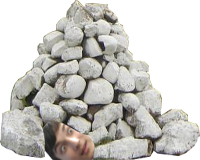## Mackenzie New Year's Challenge P6 - Rocks

View as PDF

Points: 15 (partial)
Time limit: 1.8s
Memory limit: 256M

Author:
Problem types

Nikita has a profound collection of rocks, all of which have names. In his spare time, Nikita loves to play with his rocks. He does this by arranging his rocks in a line. He occasionally adds more rocks to the end of the line. Unfortunately, he has so many rocks, that he often forgets which rocks he already added. He is not allowed to add a rock that already exists in the line (because they're all unique!). He may also switch the position of two rocks. More importantly, the most fun part is finding the mass of a few consecutive rocks! Obviously, you get the mass of each rock from its name. The name of each rock is unique and consists only of lowercase letters. The longest name Nikita will assign a rock is 100 letters long. The mass of a rock is the sum of the letters in its name, where a = 1, b = 2, c = 3 … z = 26. E.g. rock will have a mass of 47.#### Input Specification

You will executecommands. There are 5 types of commands, in the following format:

• A R - add rock R to the end of the line, R is a string – the name of the rock, output Can't add R if rock R already exists in the line.
• S X Y - swap the position of rocks X and Y – X and Y are both strings – the name of the two rocks, it is guaranteed that both X and Y exist in the line.
• M X Y - output the mass of rocks in between (inclusive) the rocks X and Y. Both the rocks are guaranteed to exist in the line.
• R X Y - replace rock X with new rock Y – X and Y are both strings. X is guaranteed to exist in the line and Y is guaranteed to not exist in the line.
• N - output the number of rocks currently in the line.

The nextlines contain these 1 lined commands. There will be at mostrocks in the line at a time.

##### For 5% of the points##### For 20% of the points, whereis number of commands., whereis the maximum number of rocks in the line., whereis the maximum length of a name Nikita will assign a rock.

##### For 50% of the points, whereis number of commands., whereis the maximum number of rocks in the line., whereis the maximum length of a name Nikita will assign a rock.

##### For 100% of the points, whereis number of commands., whereis the maximum number of rocks in the line., whereis the maximum length of a name Nikita will assign a rock.

Note: You will have to use fast input/output methods to pass large test cases.

#### Output Specification

Output depends on the commands in input. See the input specification. All output for each command goes on its own separate line.

#### Sample Input

12
A a
A b
A c
M a c
M b c
S a c
M b a
R c d
M d b
A c
A d
N

#### Sample Output

6
5
3
6
Can't add d
4

#### Explanation for Sample Output

After the first 3 commands, the rocks that exist in the line are a, b, c, in that order. The mass of a to c is a + b + c = 1 + 2 + 3 = 6. The mass of b, c is 2 + 3 = 5. The position of a and b are swapped so that the line is now c, b, a. The mass of b and a is 2 + 1 = 3. Rock c is taken out and replaced with rock d so the line becomes d, b, a. The mass of d and b is 4 + 2 = 6. Rock c gets added successfully into the line, which is now d, b, a, c. Rock d can't get added since it already exists. Finally, there are 4 rocks in the line at the end.

## Comments

• commented on Sept. 29, 2016, 10:10 p.m. edited

Image link is broken

• commented on Sept. 29, 2016, 11:02 p.m.

Fixed

• commented on Dec. 30, 2015, 10:38 a.m.

Is the first command guaranteed to be A or N? No other commands are possible.

• commented on Dec. 30, 2015, 11:26 a.m. edited

It's guaranteed to be A.